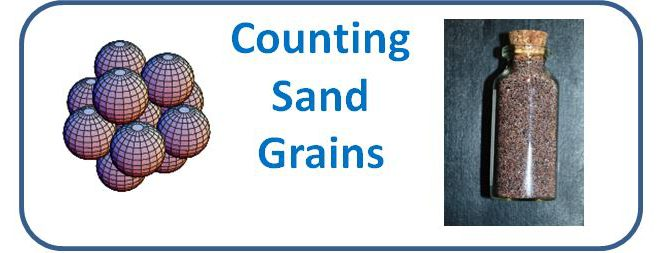# Counting Sand Grains

Did you ever wonder how many grains of sand were on a beach?  Or perhaps the simpler question of how many grains of sand fit into a 20 ml collecting vial like the one in the cover photo?   I imagine you probably have not, but I did.

To get started, we know that 20 ml is equal to 20 cubic centimeters.  Now we also will make three assumptions:

• assume a medium-grained, well-sorted sand with a grain diameter of 0.33 mm (all grains equal)
• assume well rounded spherical grains
• assume hexagonal close packing (HCP) of the grains where alternating layers of grains sit on top of each other. Each sphere will touch 12 others, 6 within its layer and 3 in the layers above and below.  With this packing of grains, the grains will comprise 60% of the volume in the vial.  The rest will be pore space.  Put another way, the porosity in the sand will be 40%

Now we are ready to do some math.  There are multiple ways to perform this calculation, but let’s work it two different ways.   Why, you ask, do we do it two ways?  Well, because we can.  And because math with a purpose is fun!

First method:  Convert the problem into a standard cube and work only with length dimensions.  This simple method requires only the first two assumptions.

• Each edge of a cube with a volume of 20 cubic centimeters will be 2.714 cm long. (or the cube root of 20).  Yes, I do know I violated significant digit rules with this conversion and others).
• This allows for 82.2 cubes with a diameter of 0.33 mm to align along each edge (27.14 mm/0.33 mm = 82.2)
• 2 x 82.2 x82.2 is ~555,000
• There are about 555,000 tiny cubes with an edge length of 0.33 mm in a 20 cubic centimeter or 20 ml volume. Each could hold one spherical grain if they were stacked directly above each other.

Of course, they would never stack that way so …

Second method:  Construct the problem to account for the spherical grain shape that is more commensurate with actual sand and incorporating the third assumption.

• The volume of a sphere is equal to 4/3 r3 where π is 3.1416 and r is the sphere radius (in this case 0.166 mm).  The volume of one sand grain is therefore 0.192 cubic millimeters.
• There are 20,000 cubic millimeters in 20 cubic centimeters (or ml).
• So there is room for ~1,040,000 sand grains in that volume (20,000/0.0192).
• BUT wait, we assumed HCP for these grains and 40% porosity. Therefore, we must multiply our calculated grain count by the percentage of the volume that would be occupied by grains.   (60% of 1,040,000 = 624,000.

The larger number (624,000 grains in a 20ml volume) is likely a more accurate assessment because it incorporates the concept that the grains will fill the space more effectively that just sitting atop the center of the one below.  In reality all the grains are not the same size or shape and these size variations generally allow for closer packing as smaller grains settle into spaces between larger grains.  However, I do not think I plan to count sand grains in any of my 20 ml sample vials to verify this.20 ml vial of medium-grained garnet-rich sand from Hamlin Beach on Lake Ontario. Imagine that, over half a million grains of sand!

Remember, sand grain size ranges from 0.06mm (very fine) to 2.0 mm (very coarse).  There would be a large difference in the number of sand grains in your 20 ml sample vial depending on the grain size of the sand.  I will let you calculate this difference.  Anyone who does can report on their results at the next club meeting.

I will also leave it to you to calculate how many 20ml vials it would take to remove all the sand from your favorite beach!

Who said math was useless?